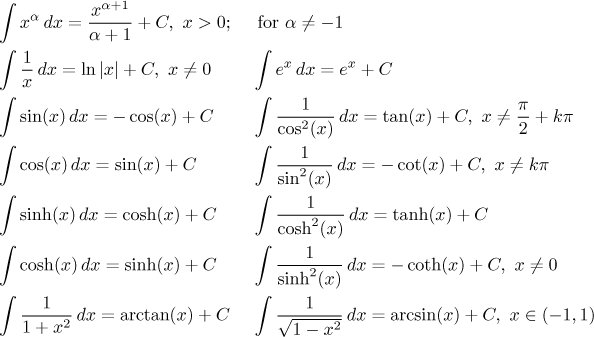# Box "elementary integrals"

The most important elementary integrals (which we should remember) areNote that the elementary integrals can be used only exactly as written, any modification means that the formula no longer works. For instance, we know that an antiderivative of cos(x) is sin(x), but this rule does not apply to things like cos(2x), cos(x2), cos2(x), etc (for instance, antiderivative of cos(2x) is definitely not sin(2x)). Such integrals have to be handled using integration techniques (often using substitution).

If we can use study materials and/or books, the list gets longer. Here you find some integrals that should be in a typical list of integrals. Since the lists differ, it is a good idea to familiarize yourself with them, so that you have a vague idea concerning what types of integrals are (and are not) in there. A student that starts every integration problem by leafing through a thick book will not have enough time to solve those that he does not find. Moreover, one rarely gets elementary integrals, more often one has to transform the given integral into an elementary ones. If you do not know them, you do not know where to go with your integral.